Anúncio

# GENCHEM1-nomenclature.pptx

21 de Mar de 2023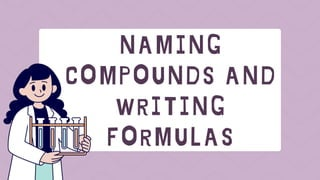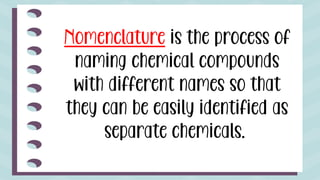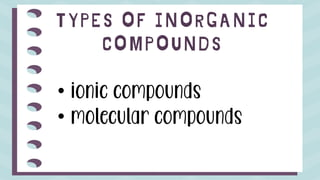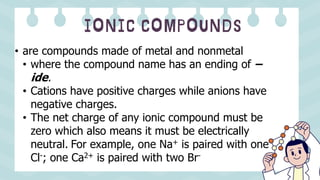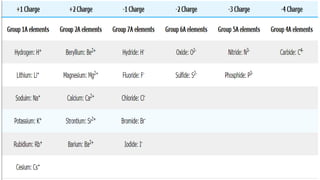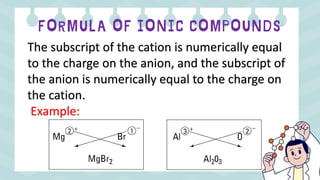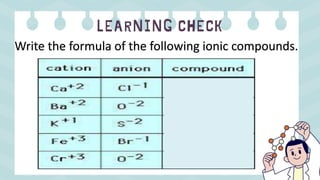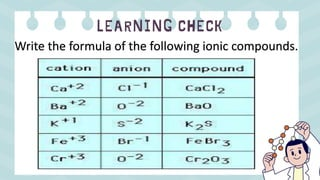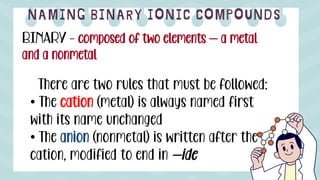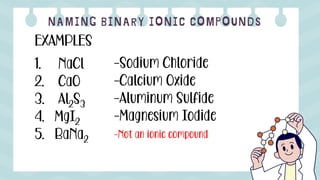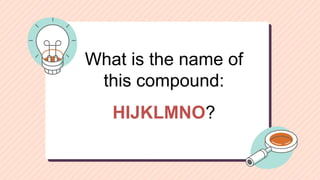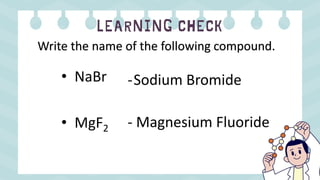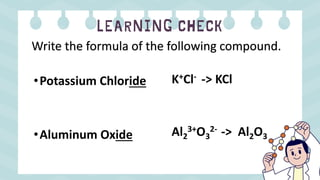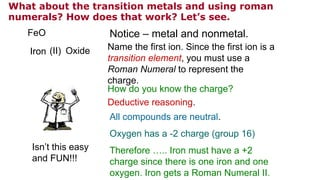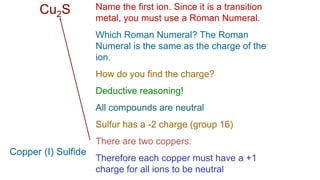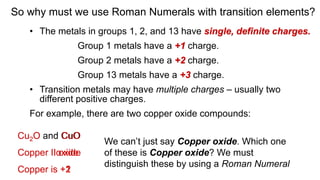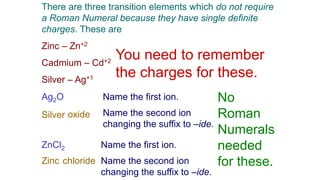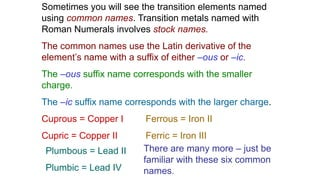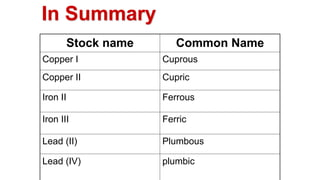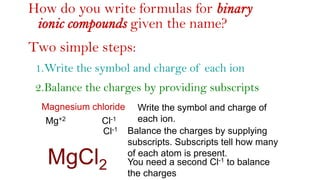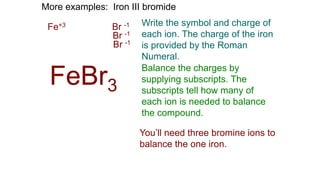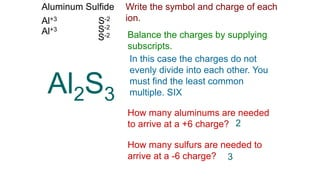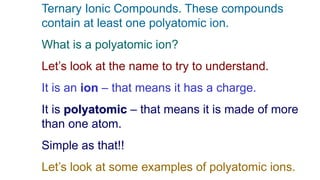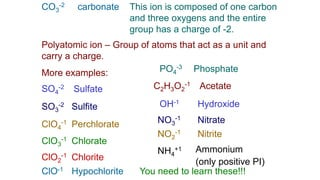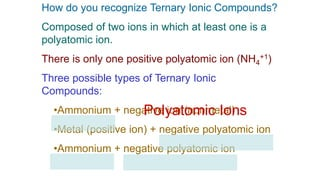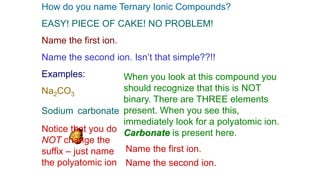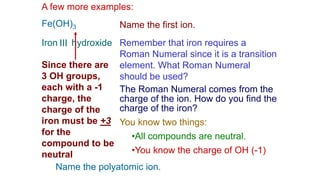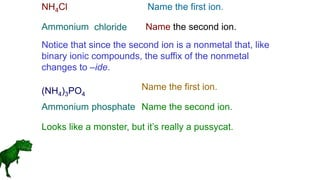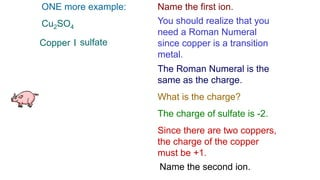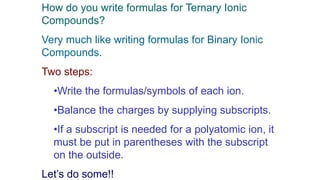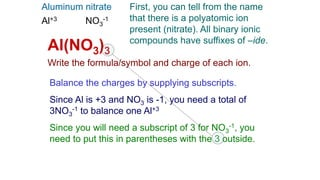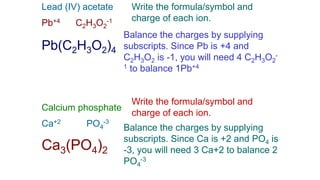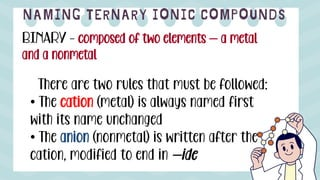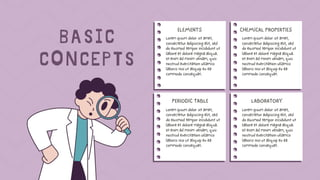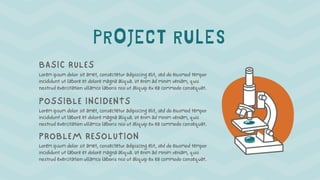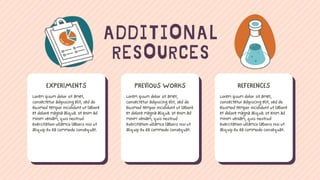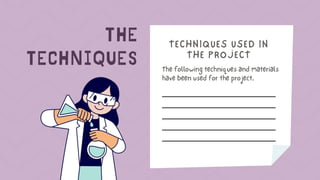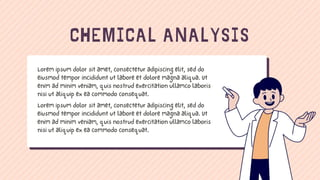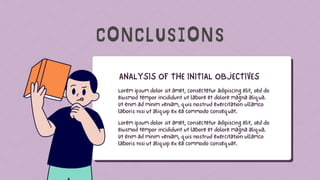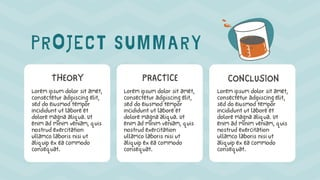1 de 43
Anúncio

### GENCHEM1-nomenclature.pptx

1. Nomenclature is the process of naming chemical compounds with different names so that they can be easily identified as separate chemicals.
2. • ionic compounds • molecular compounds
3. • are compounds made of metal and nonmetal • where the compound name has an ending of – ide. • Cations have positive charges while anions have negative charges. • The net charge of any ionic compound must be zero which also means it must be electrically neutral. For example, one Na+ is paired with one Cl-; one Ca2+ is paired with two Br-
4. The subscript of the cation is numerically equal to the charge on the anion, and the subscript of the anion is numerically equal to the charge on the cation. Example:
5. Write the formula of the following ionic compounds.
6. Write the formula of the following ionic compounds.
7. BINARY - composed of two elements – a metal and a nonmetal There are two rules that must be followed: • The cation (metal) is always named first with its name unchanged • The anion (nonmetal) is written after the cation, modified to end in –ide
8. EXAMPLES 1. NaCl 2. CaO 3. Al2S3 4. MgI2 5. BaNa2 -Sodium Chloride -Calcium Oxide -Aluminum Sulfide -Magnesium Iodide -Not an ionic compound
9. What is the name of this compound: HIJKLMNO?
10. Write the name of the following compound. • NaBr • MgF2 -Sodium Bromide - Magnesium Fluoride
11. Write the formula of the following compound. •Potassium Chloride •Aluminum Oxide K+Cl- -> KCl Al2 3+O3 2- -> Al2O3
12. What about the transition metals and using roman numerals? How does that work? Let’s see. FeO Notice – metal and nonmetal. Name the first ion. Since the first ion is a transition element, you must use a Roman Numeral to represent the charge. How do you know the charge? Deductive reasoning. All compounds are neutral. Oxygen has a -2 charge (group 16) Therefore ….. Iron must have a +2 charge since there is one iron and one oxygen. Iron gets a Roman Numeral II. Iron (II) Oxide Isn’t this easy and FUN!!!
13. Cu2S Name the first ion. Since it is a transition metal, you must use a Roman Numeral. Which Roman Numeral? The Roman Numeral is the same as the charge of the ion. How do you find the charge? Deductive reasoning! All compounds are neutral Sulfur has a -2 charge (group 16) There are two coppers. Therefore each copper must have a +1 charge for all ions to be neutral Copper (I) Sulfide
14. 1. MnO2 2. CuCl2 3. FeI2 4. PbCl4 5. FeS - Manganese (IV) oxide - Copper (II) chloride - Iron (II) iodide - Lead (IV) chloride - Iron (II) sulfide
15. So why must we use Roman Numerals with transition elements? • The metals in groups 1, 2, and 13 have single, definite charges. Group 1 metals have a +1 charge. Group 2 metals have a +2 charge. Group 13 metals have a +3 charge. • Transition metals may have multiple charges – usually two different positive charges. For example, there are two copper oxide compounds: Cu2O and CuO We can’t just say Copper oxide. Which one of these is Copper oxide? We must distinguish these by using a Roman Numeral Cu2O Copper I oxide Copper is +1 CuO Copper II oxide Copper is +2
16. There are three transition elements which do not require a Roman Numeral because they have single definite charges. These are Zinc – Zn+2 Cadmium – Cd+2 Silver – Ag+1 You need to remember the charges for these. Ag2O Name the first ion. Silver Name the second ion changing the suffix to –ide. oxide ZnCl2 Name the first ion. Name the second ion changing the suffix to –ide. Zinc chloride No Roman Numerals needed for these.
17. Sometimes you will see the transition elements named using common names. Transition metals named with Roman Numerals involves stock names. The common names use the Latin derivative of the element’s name with a suffix of either –ous or –ic. The –ous suffix name corresponds with the smaller charge. The –ic suffix name corresponds with the larger charge. Cuprous = Copper I Cupric = Copper II Ferrous = Iron II Ferric = Iron III Plumbous = Lead II Plumbic = Lead IV There are many more – just be familiar with these six common names.
18. Stock name Common Name Copper I Cuprous Copper II Cupric Iron II Ferrous Iron III Ferric Lead (II) Plumbous Lead (IV) plumbic In Summary
19. How do you write formulas for binary ionic compounds given the name? Two simple steps: 1.Write the symbol and charge of each ion 2.Balance the charges by providing subscripts Magnesium chloride Write the symbol and charge of each ion. Mg+2 Cl-1 Balance the charges by supplying subscripts. Subscripts tell how many of each atom is present. You need a second Cl-1 to balance the charges Cl-1 MgCl2
20. More examples: Iron III bromide Write the symbol and charge of each ion. The charge of the iron is provided by the Roman Numeral. Fe+3 Br -1 Balance the charges by supplying subscripts. The subscripts tell how many of each ion is needed to balance the compound. You’ll need three bromine ions to balance the one iron. Br -1 Br -1 FeBr3
21. Aluminum Sulfide Write the symbol and charge of each ion. Al+3 S-2 Balance the charges by supplying subscripts. In this case the charges do not evenly divide into each other. You must find the least common multiple. SIX How many aluminums are needed to arrive at a +6 charge? How many sulfurs are needed to arrive at a -6 charge? 2 3 Al+3 S-2 S-2 Al2S3
22. Ternary Ionic Compounds. These compounds contain at least one polyatomic ion. What is a polyatomic ion? Let’s look at the name to try to understand. It is an ion – that means it has a charge. It is polyatomic – that means it is made of more than one atom. Simple as that!! Let’s look at some examples of polyatomic ions.
23. CO3 -2 carbonate This ion is composed of one carbon and three oxygens and the entire group has a charge of -2. Polyatomic ion – Group of atoms that act as a unit and carry a charge. More examples: SO4 -2 Sulfate SO3 -2 Sulfite ClO4 -1 Perchlorate ClO3 -1 Chlorate ClO2 -1 Chlorite ClO-1 Hypochlorite PO4 -3 Phosphate C2H3O2 -1 Acetate OH-1 Hydroxide NO3 -1 Nitrate NO2 -1 Nitrite You need to learn these!!! NH4 +1 Ammonium (only positive PI)
24. How do you recognize Ternary Ionic Compounds? Composed of two ions in which at least one is a polyatomic ion. There is only one positive polyatomic ion (NH4 +1) Three possible types of Ternary Ionic Compounds: •Ammonium + negative ion (nonmetal) •Metal (positive ion) + negative polyatomic ion •Ammonium + negative polyatomic ion Polyatomic Ions
25. How do you name Ternary Ionic Compounds? EASY! PIECE OF CAKE! NO PROBLEM! Name the first ion. Name the second ion. Isn’t that simple??!! Examples: Na2CO3 When you look at this compound you should recognize that this is NOT binary. There are THREE elements present. When you see this, immediately look for a polyatomic ion. Carbonate is present here. Name the first ion. Sodium Name the second ion. carbonate Notice that you do NOT change the suffix – just name the polyatomic ion
26. A few more examples: Fe(OH)3 Name the first ion. Iron Remember that iron requires a Roman Numeral since it is a transition element. What Roman Numeral should be used? The Roman Numeral comes from the charge of the ion. How do you find the charge of the iron? You know two things: •All compounds are neutral. •You know the charge of OH (-1) Since there are 3 OH groups, each with a -1 charge, the charge of the iron must be +3 for the compound to be neutral III Name the polyatomic ion. hydroxide
27. NH4Cl Name the first ion. Ammonium Name the second ion. chloride Notice that since the second ion is a nonmetal that, like binary ionic compounds, the suffix of the nonmetal changes to –ide. (NH4)3PO4 Name the first ion. Name the second ion. Ammonium phosphate Looks like a monster, but it’s really a pussycat.
28. ONE more example: Cu2SO4 Name the first ion. Copper You should realize that you need a Roman Numeral since copper is a transition metal. The Roman Numeral is the same as the charge. What is the charge? The charge of sulfate is -2. Since there are two coppers, the charge of the copper must be +1. I sulfate Name the second ion.
29. How do you write formulas for Ternary Ionic Compounds? Very much like writing formulas for Binary Ionic Compounds. Two steps: •Write the formulas/symbols of each ion. •Balance the charges by supplying subscripts. •If a subscript is needed for a polyatomic ion, it must be put in parentheses with the subscript on the outside. Let’s do some!!
30. Aluminum nitrate First, you can tell from the name that there is a polyatomic ion present (nitrate). All binary ionic compounds have suffixes of –ide. Write the formula/symbol and charge of each ion. Al+3 NO3 -1 Balance the charges by supplying subscripts. Since Al is +3 and NO3 is -1, you need a total of 3NO3 -1 to balance one Al+3 Since you will need a subscript of 3 for NO3 -1, you need to put this in parentheses with the 3 outside. Al(NO3)3
31. Lead (IV) acetate Write the formula/symbol and charge of each ion. Pb+4 C2H3O2 -1 Balance the charges by supplying subscripts. Since Pb is +4 and C2H3O2 is -1, you will need 4 C2H3O2 - 1 to balance 1Pb+4 Pb(C2H3O2)4 Calcium phosphate Write the formula/symbol and charge of each ion. Balance the charges by supplying subscripts. Since Ca is +2 and PO4 is -3, you will need 3 Ca+2 to balance 2 PO4 -3 Ca+2 PO4 -3 Ca3(PO4)2
32. BINARY - composed of two elements – a metal and a nonmetal There are two rules that must be followed: • The cation (metal) is always named first with its name unchanged • The anion (nonmetal) is written after the cation, modified to end in –ide

### Notas do Editor

1. Generally, there are two types of inorganic compounds that can be formed: ionic compounds and molecular compounds.
2. Generally, there are two types of inorganic compounds that can be formed: ionic compounds and molecular compounds.
Anúncio